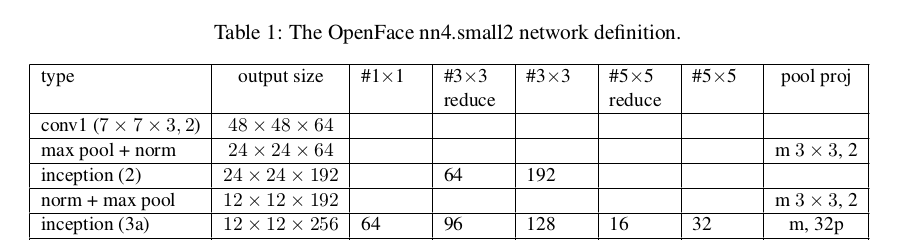/ openface Public

# nn4.small2.v1.t7 Incorrect Layer Error#349

Closed
opened this issue Feb 28, 2018 · 3 comments
Closed

# nn4.small2.v1.t7 Incorrect Layer Error #349

opened this issue Feb 28, 2018 · 3 comments

### Context of the issue.

I'm getting discrepancies on your `nn4.small2.v1.t7` model related to the Inception(3a) layer. The actual model posted to your website does not match up with your GitHub definition.

### Expected behavior.

According to your definition, on line 67 you have `pool = nn.SpatialMaxPooling(3, 3, 1, 1, 1, 1)`, indicating parameters:

``````kW=3
kH=3
dW=1
dH=1
pW=1
pH=1
``````

### Actual behavior.

When I actually load and print the model from your website on Torch, I get different numbers:

``````nn.Sequential {
[input -> (1) -> (2) -> (3) -> (4) -> (5) -> (6) -> (7) -> (8) -> (9) -> (10) -> (11) -> (12) -> (13) -> (14) -> (15) -> (16) -> (17) -> (18) -> (19) -> (20) -> (21) -> (22) -> (23) -> (24) -> (25) -> (26) -> output]
(1): nn.SpatialConvolutionMM(3 -> 64, 7x7, 2,2, 3,3)
(2): nn.SpatialBatchNormalization (4D) (64)
(3): nn.ReLU
(4): nn.SpatialMaxPooling(3x3, 2,2, 1,1)
(5): nn.SpatialCrossMapLRN
(6): nn.SpatialConvolutionMM(64 -> 64, 1x1)
(7): nn.SpatialBatchNormalization (4D) (64)
(8): nn.ReLU
(9): nn.SpatialConvolutionMM(64 -> 192, 3x3, 1,1, 1,1)
(10): nn.SpatialBatchNormalization (4D) (192)
(11): nn.ReLU
(12): nn.SpatialCrossMapLRN
(13): nn.SpatialMaxPooling(3x3, 2,2, 1,1)
(14): nn.Inception @ nn.DepthConcat {
input
|`-> (1): nn.Sequential {
|      [input -> (1) -> (2) -> (3) -> (4) -> (5) -> (6) -> output]
|      (1): nn.SpatialConvolution(192 -> 96, 1x1)
|      (2): nn.SpatialBatchNormalization (4D) (96)
|      (3): nn.ReLU
|      (4): nn.SpatialConvolution(96 -> 128, 3x3, 1,1, 1,1)
|      (5): nn.SpatialBatchNormalization (4D) (128)
|      (6): nn.ReLU
|    }
|`-> (2): nn.Sequential {
|      [input -> (1) -> (2) -> (3) -> (4) -> (5) -> (6) -> output]
|      (1): nn.SpatialConvolution(192 -> 16, 1x1)
|      (2): nn.SpatialBatchNormalization (4D) (16)
|      (3): nn.ReLU
|      (4): nn.SpatialConvolution(16 -> 32, 5x5, 1,1, 2,2)
|      (5): nn.SpatialBatchNormalization (4D) (32)
|      (6): nn.ReLU
|    }
|`-> (3): nn.Sequential {
|      [input -> (1) -> (2) -> (3) -> (4) -> output]
|      (1): nn.SpatialMaxPooling(3x3, 2,2)

.
.
.
``````

Where nn.SpatialMaxPooling:

``````kW = 3
kH = 3
dW = 2
dH = 2
``````

The error may be occuring on other layers too, but I haven't had time to check yet.

### Steps to reproduce.

``````#!/usr/bin/env th
require 'torch'
require 'nn'
require 'dpnn'

torch.setdefaulttensortype('torch.FloatTensor')

print(net)

``````

### OS and hardware information.

• Operating system: Ubuntu 16.04 LTS
• Torch version: 7

### bamos commented Feb 28, 2018

 Great spot, thanks for letting me know! I'd merge in a PR making the model definition consistent with the pretrained model.

### k22jung commented Feb 28, 2018

 I'm not quite sure if it's an issue regarding the definition on GitHub or your website model. From what I understand, the definition corresponds with your paper.As shown, the input size for 3a is (12 x 12 x 192) from `norm + max pool` and 3a's output is (12 x 12 x 256). Using the def's numbers and formula `H = (H1 - F + 2P)/S +1`, it gives the correct height of the output. You would get `H = 5.5 ~= 6 != 12` if you were to use the model's parameters. However, if the model is an issue, I'm not sure how it's able to do training and forward pass correctly if the parameters don't match up.

### k22jung commented Mar 1, 2018

 I was able to fix the parameters of 3a and the other layers' `nn.SpatialMaxPooling` to what you have in the def, and it seemed to give good results. But I think `nn.SpatialLPPooling` of the other layers require `padH = padW =1`, because sizes don't seem to work with what you have in the definition.

pushed a commit that referenced this issue Mar 2, 2018
``` Mention inconsistencies between the pre-trained models and the model … ```
``` bb377aa ```
`…definitions for #351 and #349`
pushed a commit to liuwanjin365/openface that referenced this issue Apr 28, 2021
``` Mention inconsistencies between the pre-trained models and the model … ```
``` ab314ca ```
`…definitions for cmusatyalab#351 and cmusatyalab#349`